# Polyhedral skeletal electron pair theory

﻿
Polyhedral skeletal electron pair theory

In chemistry the polyhedral skeletal electron pair theory provides electron counting rules useful for predicting the structures of clusters such as borane and carborane clusters. The electron counting rules were originally formulated by Kenneth Wade and were further developed by D. M. P. Mingos and others; they are sometimes known as Wade's rules or the Wade/Mingos rules. The rules are based on a molecular orbital treatment of the bonding.. Recently these rules are extended and unified for macropolyhedral boranes, metallocenes and borides. The unified electron counting rules are called Jemmis mno rules.

## Predicting structures of cluster compounds

Different rules (4n, 5n, or 6n) are invoked depending on the number of electrons per vertex.

The 4n rules are reasonably accurate in predicting the structures of clusters having about 4 electrons per vertex, as is the case for many boranes and carboranes. For such clusters, the structures are based on deltahedra, which are polyhedra in which every face is triangular. The 4n clusters are classified as closo-, nido-, arachno- or hypho-, based on whether they represent a complete (closo-) deltahedron, or a deltahedron that is missing one (nido-), two (arachno-) or three (hypho) vertices.

However, hypho clusters are relatively uncommon due to the fact that the electron count is high enough to start to fill antibonding orbitals and destabilize the 4n structure. If the electron count is close to 5 electrons per vertex, the structure often changes to one governed by the 5n rules, which are based on 3-connected polyhedra.

As the electron count increases further, the structures of clusters with 5n electron counts become unstable, so the 6n rules can be implemented. The 6n clusters have structures that are based on rings.

A molecular orbital treatment can be used to rationalize the bonding of cluster compounds of the 4n, 5n, and 6n types.

### 4n rules

The following polyhedra are the basis for the 4n rules; each of these have triangular faces. The number of vertices in the cluster determines what polyhedron the structure is based on.

Number of vertices Polyhedron
4 Tetrahedron
5 Trigonal bipyramid
6 Octahedron
7 Pentagonal bipyramid
8 D2d (trigonal) dodecahedron
9 Tricapped trigonal prism
10 Bicapped square antiprism
12 Icosahedron (bicapped pentagonal antiprism)

Using the electron count, the predicted structure can be found. n is the number of vertices in the cluster. The 4n rules are enumerated in the following table.

Electron count Name Predicted structure
4n – 2 Bicapped closo n–2 vertex closo polyhedron with 2 capped faces
4n Capped closo n–1 vertex closo polyhedron with 1 face capped
4n + 2 Closo Closo polyhedron with n vertices
4n + 4 Nido n + 1 vertex closo polyhedron with 1 missing vertex
4n + 6 Arachno n + 2 vertex closo polyhedron with 2 missing vertices
4n + 8 Hypho n + 3 vertex closo polyhderon with 3 missing vertices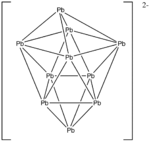Pb102–

When counting electrons for each cluster, the number of valence electrons is enumerated. For each transition metal present, 10 electrons are subtracted from the total electron count. For example, in Rh6(CO)16 the total number of electrons would be 6(9) + 16(2) - 6(10) = 86 – 6(10) = 26. Therefore, the cluster is a closo polyhedron because n = 6, with 4n + 2 = 26.S42+

Other rules may be considered when predicting the structure of clusters:

1. For clusters consisting mostly of transition metals, any main group elements present are often best counted as ligands or interstitial atoms, rather than vertices.
2. Larger and more electropositive atoms tend to occupy vertices of high connectivity and smaller more electronegative atoms tend to occupy vertices of low connectivity.
3. In the special case of boron hydride clusters, each boron connected to 3 or more vertices has one terminal hydride, while a boron connected to 2 other vertices has 2 terminal hydrogens. If more hydrogens are present, they are placed in open face positions to even out the coordination number of the vertices.
4. For the special case of transition metal clusters, ligands are added to the metal centers to give the metals reasonable coordination numbers, and if any hydrogen atoms are present they are placed in bridging positions to even out the coordination numbers of the vertices.

In general, closo structures with n vertices are n-vertex polyhedra.

To predict the structure of a nido cluster, the closo cluster with n + 1 vertices is used as a starting point; if the cluster is composed of small atoms a high connectivity vertex is removed, while if the cluster is composed of large atoms a low connectivity vertex is removed.

To predict the structure of an arachno cluster, the closo polyhedron with n + 2 vertices is used as the starting point, and the n+1 vertex nido complex is generated by following the rule above; a second vertex adjacent to the first is removed if the cluster is composed of mostly small atoms, a second vertex not adjacent to the first is removed if the cluster is composed mostly of large atoms.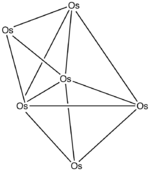Os6(CO)18, carbonyls omitted

Example: Pb102–

Electron count: 10(Pb) + 2 (for the negative charge) = 10(4) + 2 = 42 electrons.
Since n = 10, 4n + 2 = 42, so the cluster is a closo bicapped square antiprism.

Example: S42+

Electron count: 4(S) – 2 (for the positive charge) = 4(6) – 2 = 22 electrons.
Since n = 4, 4n + 6 = 22, so the cluster is arachno.
Starting from an octahedron, a vertex of high connectivity is removed, and then a non-adjacent vertex is removed.

Example: Os6(CO)18

Electron count: 6(Os) + 18(CO) – 60 (for 6 osmium atoms) = 6(8) + 18(2) – 60 = 24
Since n = 6, 4n = 24, so the cluster is capped closo.
Starting from a trigonal bipyramid, a face is capped. The carbonyls have been omitted for clarity.B5H54–, hydrogens omitted

Example: B5H54–

Electron count: 5(B) + 5(H) + 4 (for the negative charge) = 5(3) + 5(1) + 4 = 24
Since n = 5, 4n + 4 = 24, so the cluster is nido.
Starting from an octahedron, one of the vertices is removed.

The rules are useful in also predicting the structure of carboranes. Example: C2B7H13

Electron count = (2×4) + (3×7) + (13×1) = 42
Since n in this case is 9, 4n + 6 = 42, the cluster is arachno

The bookkeeping for deltahedral clusters is sometimes carried out by counting skeletal electrons instead of the total number of electrons. The skeletal orbital (electron pair) and skeletal electron counts for the four types of deltahedral clusters are:

• n-vertex closo: (n+1) skeletal orbitals, (2n+2) skeletal electrons
• n-vertex nido: (n+2) skeletal orbitals, (2n+4) skeletal electrons
• n-vertex arachno: (n+3) skeletal orbitals, (2n+6) skeletal electrons
• n-vertex hypho: (n+4) skeletal orbitals, (2n+8) skeletal electrons

The skeletal electron counts are determined by summing the total of the following number of electrons:

• 2 from each BH unit
• 3 from each CH unit
• 1 from each additional hydrogen atom (over and above the ones on the BH and CH units)
• the anionic charge electrons

### 5n rules

As discussed previously, the 4n rule mainly deals with clusters with electron counts of 4n+k, in which approximately 4 electrons are on each vertex. As more electrons are added per vertex, the number of the electrons per vertex approaches 5. Rather than adopting structures based on deltahedra, the 5n-type clusters have structures based on a different series of polyhedra known as the 3-connected polyhedra, in which each vertex is connected to 3 other vertices. The common types of 3-connected polyhedra are listed below.5n cluster: P45n+3 cluster: P4S35n+6 cluster: P4O6
Number of vertices Type of 3-connected polyhedron
4 Tetrahedron
6 Trigonal prism
8 Cube
10 Pentagonal prism
12 D2d Pseudo-octahedron

The 5n rules are as follows.

Total electron count Predicted structure
5n n-vertex 3-connected polyhedron
5n+1 n–1 vertex 3-connected polyhedron with one vertex inserted into an edge
5n+2 n–2 vertex 3-connected polyhedron with two vertexes inserted into edges
5n+k n-k vertex 3-connected polyhedron with k vertexes inserted into edges

Example: P4

Electron count: 4(P)= 4(5)= 20
It is 5n structure with n=4, so it is tetrahedral

Example: P4S3

Electron count 4(P)+ 3(S)= 4(5)+3(6)= 38
It is 5n+3 structure with n=7. Three vertices are inserted into edges

Example: P4O6

Electron count 4(P)+ 6(O)= 4(5)+ 6(6)= 56
It is 5n+6 structure with n=10. Six vertices are inserted into edges

### 6n rules

As more electrons are added to a 5n cluster, the number of electrons per vertex approaches 6. Instead of adopting structures based on 4n or 5n rules, the clusters tend to have structures goverened by the 6n rules, which are based on rings. The rules for the 6n structures are as follows.

Total electron count Predicted structure
6n–k n-membered ring with k/2 trans-annular bonds
6n–4 n-membered ring with 2 trans-annular bonds
6n–2 n-membered ring with 1 trans-annular bond
6n n-membered ring
6n+2 n-membered chain (n-membered ring with 1 broken bond)

Example: S8

Electron count = S(8) = 6(8) = 48 electrons.
Since n = 8, 6n = 48, so the cluster is an 8 membered ring.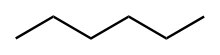6n+2 cluster: hexane

Hexane (C6H14)

Electron count = C(6)+H(14) = 6(6) + 1(14)=38
Since n=6, 6n=36 and 6n+2=38 so the cluster is a 6 membered chain.

### Isolobal vertex units

Provided a vertex unit is isolobal with BH then it can, in principle at least, be substituted for a BH unit, even though that BH and CH are not isoelectronic. The CH+ unit is isolobal, hence the reason why the rules are applicable to carboranes.
This can be explained due to a frontier orbital treatment. Additionally there are isolobal transition metal units. For example Fe(CO)3 provides 2 electrons. The derivation of this is briefly as follows:

• Fe has 8 valence electrons.
• Each carbonyl group is a net 2 electron donor after the internal σ and π bonding are taken into account making 14 electrons.
• 3 pairs are considered to be involved in Fe – CO σ-bonding and 3 pairs are involved in π back bonding from Fe to CO reducing the 14 to 2.

## Bonding in cluster compounds

### Polyhedron

B2H6Molecular-orbital (MO) diagram of B2H6. Atoms and their corresponding orbitals are colored the same. Green MOs symbolize bonding, while red symbolize anti-bonding.

The bonding in diborane is best described by treating each B as sp3 hybridized. Two sp3 hybrid orbitals on each boron form the bonds to the terminal hydrogens. The remaining sp3 orbitals create the bonds with the bridging hydrogens. Because the angles in the diborane structure are not tetrahedral the orbitals also likely contain some sp2 character.

Closo-B6H62–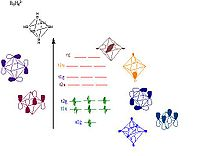MO diagram of B6H62– showing the orbitals responsible for forming the cluster. Pictorial representations of the orbitals are shown; the MO sets of T and E symmetry will each have two or one additional pictorial representation, respectively, that are not shown here.

The boron atoms lie on each vertex of the octahedron and are sp hybridized. One sp hybrid radiates away from the structure forming the bond with the hydrogen atom. The other sp hybrid radiates into the center of the structure forming a large bonding molecular orbital at the center of the cluster. The remaining two unhybridized orbitals lie along the tangent of the sphere like structure creating more bonding and antibonding orbitals between the boron vertices.

The orbital diagram breaks down as follows

The 18 framework molecular orbitals, (MOs), derived from the 18 boron atomic orbitals are:

• 1 bonding MO at the center of the cluster and 5 antibonding MOs from the 6 sp radial hybrid orbitals
• 6 bonding MOs and 6 antibonding MOs from the 12 tangential p orbitals.

The total skeletal bonding orbitals is therefore 7, i.e. (n+1).

Main group atom clusters The bonding in other main group cluster compounds follow similar rules as those described for the boron cluster bonding. The atoms at the vertex hybridize in a way which allows the lowest energy structure to form.

The 18 framework molecular orbitals, (MOs), derived from the 18 boron atomic orbitals are:

• 1 bonding MO at the center of the cluster and 5 antibonding MOs from the 6 sp radial hybrid orbitals
• 6 bonding MOs and 6 antibonding MOs from the 12 tangential p orbitals.

The total skeletal bonding orbitals is therefore 7, i.e. (n+1).

### Transition metal clusters

Transition metal clusters use the d orbitals for bonding so have up to nine bonding orbitals, instead of only the four present in boron and main group clusters. There is also more bonding flexibility in transition metal clusters depending on whether vertex metal electron pairs are involved in cluster bonding or appear as lone pairs.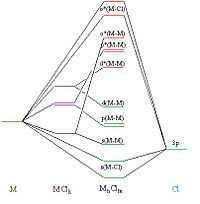General MO diagram of metal chloride structures. Green MOs represent bonding orbitals while red represent anti-bonding orbitals. The labeling on the MOs is as follows: s-sigma, p-pi, d-delta bonding, with * denoting anti-bonding interactions.

The cluster chlorides and carbonyls of transition metals will be briefly discussed here as they represent opposite ends of the spectrochemical series and show important features of the differences between transition metal clusters with different ligands. In chloride clusters the energy splitting of the valence d orbitals increases upon formation of the cluster. The number and symmetry of these orbitals are dependent upon the type and structure of each individual cluster complex. Conversely in the carbonyl clusters the energy splitting of the valence d orbitals is greater before the formation of the cluster.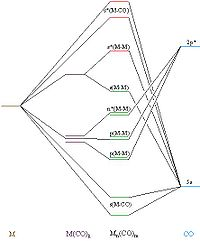General MO diagram for metal carbonyl clusters. Green MOs represent bonding orbitals while red represent anti-bonding orbitals. The labeling on the MOs is as follows:s-sigma, p-pie, d-delta bonding with * denoting anti-bonding interactions.

## Applications of cluster compounds

### Catalysis

Due to the wide variety of cluster material discovered, the applications of cluster materials lie in many fields. One major application of the metal based cluster is in catalysis. It is believed that the cluster material can serve as the model material to study heterogeneous catalyst, since it is easier to characterize the surface species and study the mechanism of the reactions that take place in the solution than those on the metal surface. Apart from the application in model study, the cluster its own can be used as effective catalyst. For instance, Pt cluster, [Pt15(CO)30]2– has been reported to catalyze the selective hydrogenation of organic compounds including olefins, ketones and aldehydes. Heteronuclear cluster H4Pt3Ru6(CO)21 catalyses various alkyne transformations. There has been general debate about whether the cluster remains intact during the catalysis or they fragment to from mononuclear species or aggregate to form nanoparticles. It is now known that examples involving all three possibilities exist and in the same reaction more than one catalyst can operate simultaneously.

### Medical treatment

Metal clusters with radioactive isotopes are considered as potential candidates for medical treatment of cancer. Compared with mononuclear rhenium complexes, the polynuclear rhenium cluster has higher density of the metal isotopes, which is beneficial to the radiotherapy, since the active materials in the treatment are the metal elements. One typical sample is the octahedral rhenium metal cluster based on the radioactive isotopes 186Re and 188Re, such as K4[{Re6-Se8}(OH)6]∙8H2O and K4[{Re6-S8}(OH)6]∙8H2O. The former does not show acute cytotoxic effect up to 50 micromoles, which is the practical concentration level of biological applications. Meanwhile, the octahedral rhenium cluster complexes can be potentially applied for photodynamic therapy, because such cluster complexes display photoluminescence in the spectral region where the photodynamic therapy is effective.

### Building blocks for mesoscale structure

Some cluster complex can function as the building block for other mesoscale materials, like porous framework, nanoparticles and mesoporous materials. It is known that [Re6Se8]2+ face-capped octahedral clusters can be used as fundamental building units to create extended arrays of clusters via secondary interaction such as hydrogen bonding and secondary metal-ligand coordination.

Wikimedia Foundation. 2010.

### Look at other dictionaries:

• Electron pair — In chemistry, an electron pair consists of two electrons that occupy the same orbital but have opposite spins.Because electrons are fermions, the Pauli exclusion principle forbids these particles from having exactly the same quantum numbers.… …   Wikipedia

• Electron counting — is a formalism used for classifying compounds and for explaining or predicting electronic structure and bonding. Many rules in chemistry rely on electron counting:*Octet rule for main group elements, especially the lighter ones such as carbon,… …   Wikipedia

• d electron count — The d electron count is a chemistry formalism used to describe the electron configuration of the valence electrons of a transition metal center in a coordination complex. The d electron count is an effective way to understand the geometry… …   Wikipedia

• Michael Mingos — David Michael Patrick Mingos FRS (born 6 August 1944) was Principal of St Edmund Hall, Oxford from 1999 to 2009, and Professor of Inorganic Chemistry in the University of Oxford. Michael Mingos is the son of Vasso Mingos, of Athens, and Rose Enid …   Wikipedia

• Cluster chemistry — In chemistry, a cluster is an ensemble of bound atoms intermediate in size between a molecule and a bulk solid. Clusters exist of diverse stoichiometries and nuclearities. For example, carbon and boron atoms form fullerene and borane clusters,… …   Wikipedia

• Olefin metathesis — or transalkylidenation is an organic reaction that entails redistribution of alkylene fragments by the scission of carbon carbon double bonds in olefins (alkenes). Its advantages include the creation of fewer sideproducts and hazardous wastes …   Wikipedia

• Transition metal carbene complex — A transition metal carbene complex is a organometallic compound featuring a divalent organic ligand. The divalent organic ligand coordinated to the metal center is called a carbene. Carbene complexes for almost all transition metals have been… …   Wikipedia

• Gilman reagent — General structure of a Gilman reagent A Gilman reagent is a lithium and copper (diorganocopper) reagent compound, R2CuLi, where R is an organic radical. These are useful because they react with organic chlorides, bromides, and iodides to replace… …   Wikipedia

• Oxidative addition — and reductive elimination are two important and related classes of reactions in organometallic chemistry. Oxidative addition is a process that increases both the oxidation state and coordination number of a metal centre. The oxidative… …   Wikipedia

• Organometallic chemistry — n Butyllithium, an organometallic compound. Four lithium atoms are shown in purple in a tetrahedron, and each lithium atom is bound to a butyl group (carbon is black, hydrogen is white). Organometallic chemistry is the study of chemical compounds …   Wikipedia